# High School Math : Cones

## Example Questions

2 Next →

### Example Question #11 : Solid Geometry

What is the volume of a cone with base radius 4, and height 6?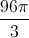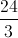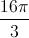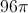Explanation:

The volume of a cone is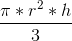, whereis the height of the cone andis the base radius.

The volume of this cone is thus: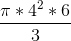=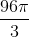### Example Question #163 : Solid Geometry

What is the surface area of a cone with a radius of 4 and a height of 3?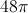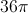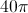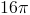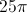Explanation:

Here we simply need to remember the formula for the surface area of a cone and plug in our values for the radius and height.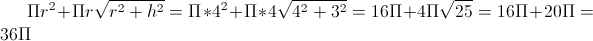### Example Question #162 : Solid Geometry

The lateral area is twice as big as the base area of a cone.  If the height of the cone is 9, what is the entire surface area (base area plus lateral area)?

81π

90π

27π

54π

81π

Explanation:

Lateral Area = LA = π(r)(l) where r = radius of the base and l = slant height

LA = 2B

π(r)(l) = 2π(r2)

rl = 2r2

l = 2r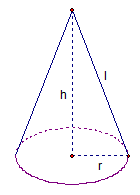From the diagram, we can see that r2 + h2 = l2.  Since h = 9 and l = 2r, some substitution yields

r2 + 92 = (2r)2

r2 + 81 = 4r2

81 = 3r2

27 = r2

B = π(r2) = 27π

LA = 2B = 2(27π) = 54π

SA = B + LA = 81π

### Example Question #12 : Solid Geometry

What is the surface area of a cone with a height of 8 and a base with a radius of 5?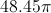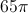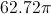Explanation:

To find the surface area of a cone we must plug in the appropriate numbers into the equation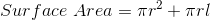whereis the radius of the base, andis the lateral, or slant height of the cone.

First we must find the area of the circle.

To find the area of the circle we plug in our radius into the equation of a circle which is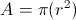This yields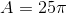.

We then need to know the surface area of the cone shape.

To find this we must use our height and our radius to make a right triangle in order to find the lateral height using Pythagorean’s Theorem.

Pythagorean’s Theorem states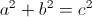Take the radius and height and plug them into the equation as a and b to yield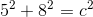First square the numbers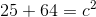After squaring the numbers add them together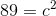Once you have the sum, square root both sides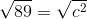After calculating we find our length is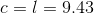Then plug the length into the second portion of our surface area equation above to get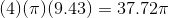Then add the area of the circle with the conical area to find the surface area of the entire figure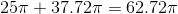The answer is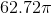.

### Example Question #1 : How To Find The Surface Area Of A Cone

What is the surface area of a cone with a radius of 6 in and a height of 8 in?

66π in2

96π in2

112π in2

36π in2

60π in2

96π in2

Explanation:

Find the slant height of the cone using the Pythagorean theorem:  r2 + h2 = s2 resulting in 62 + 82 = s2 leading to s2 = 100 or s = 10 in

SA = πrs + πr2 = π(6)(10) + π(6)2 = 60π + 36π = 96π in2

60π in2 is the area of the cone without the base.

36π in2 is the area of the base only.

### Example Question #13 : Solid Geometry

Find the surface area of a cone that has a radius of 12 and a slant height of 15.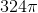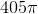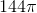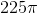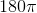Explanation:

The standard equation to find the surface area of a cone is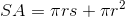wheredenotes the slant height of the cone, anddenotes the radius.

Plug in the given values forandto find the answer: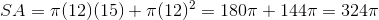### Example Question #14 : Solid Geometry

Find the surface area of the following cone.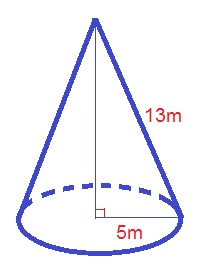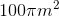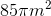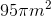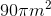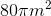Explanation:

The formula for the surface area of a cone is: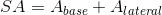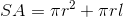whereis the radius of the cone andis the slant height of the cone.

Plugging in our values, we get: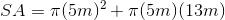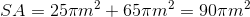### Example Question #15 : Solid Geometry

Find the surface area of the following cone.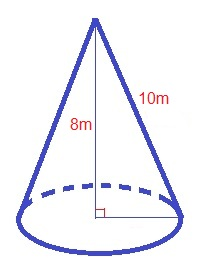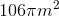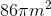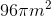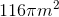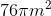Explanation:

The formula for the surface area of a cone is: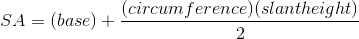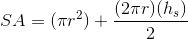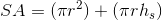Use the Pythagorean Theorem to find the length of the radius: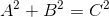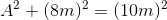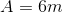Plugging in our values, we get: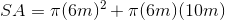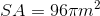### Example Question #16 : Solid Geometry

Find the surface area of the following half cone.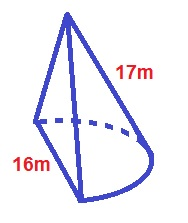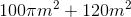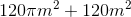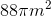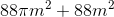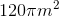Explanation:

The formula for the surface area of the half cone is: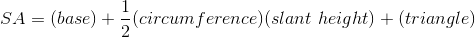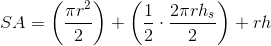Whereis the radius,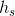is the slant height, andis the height of the cone.

Use the Pythagorean Theorem to find the height of the cone: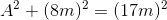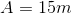Plugging in our values, we get: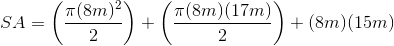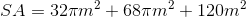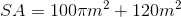2 Next →